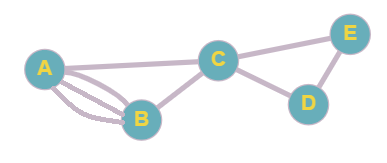# Architecture, a floor plan of a museum is shown. Draw a graph that represents the floor plan, where each vertex represents a room and an edge connects two vertices if there is a doorway between the two rooms. Is it possible to walk through the museum and pass through each doorway without going through any doorway twice? Does it depend on whether you return to the room you started at? Justify your conclusion.### Mathematical Excursions (MindTap C...

4th Edition
Richard N. Aufmann + 3 others
Publisher: Cengage Learning
ISBN: 9781305965584

#### Solutions

Chapter
Section### Mathematical Excursions (MindTap C...

4th Edition
Richard N. Aufmann + 3 others
Publisher: Cengage Learning
ISBN: 9781305965584
Chapter 5.1, Problem 29ES
Textbook Problem
673 views

## Architecture, a floor plan of a museum is shown. Draw a graph that represents the floor plan, where each vertex represents a room and an edge connects two vertices if there is a doorway between the two rooms. Is it possible to walk through the museum and pass through each doorway without going through any doorway twice? Does it depend on whether you return to the room you started at? Justify your conclusion..
To determine

To graph the floor plan. To determine if it is possible to walk through the museum and pass through each doorway without going through any doorway twice. To check if it depends on whether the ending point is same as the starting point.

### Explanation of Solution

Given information:

Given, a floor plan of a museum is shown below, in whose graph each room is represented by a vertex and a doorway between the two rooms is represented by an edge.

Calculation:

To make the graph, the rooms are labelled first as per the given information.

Using the above image, the graph can be made as:

It is possible to walk through the museum and pass through each doorway without going through any doorway twice if the graph satisfies the condition of either Euler circuit or Euler path.

An Eulerian graph (that starts and ends at the same vertex) is said to be Euler circuit if it uses every edge, but only once. (i.e. no edge is used more than once).

Euler path is defined as the path in a connected graph that has exactly two vertices of odd degree with all the remaining vertices of even degree and the path starts at one of the vertices with odd degree and ends at the other vertex with odd degree...

### Still sussing out bartleby?

Check out a sample textbook solution.

See a sample solution

#### The Solution to Your Study Problems

Bartleby provides explanations to thousands of textbook problems written by our experts, many with advanced degrees!

Get Started

Find more solutions based on key concepts
In problems 17-30, simplify each expression so that only positive exponents remain. 20.

Mathematical Applications for the Management, Life, and Social Sciences

In Exercises 9-12, use the evaluation function of your graphing utility to find the value of f at the indicated...

Applied Calculus for the Managerial, Life, and Social Sciences: A Brief Approach

In Exercises 13 and 14, determine whether y is a function of x. 9x2+4y2=49

Calculus: An Applied Approach (MindTap Course List)

Define a Type I error and a Type Il error and explain the consequences of each.

Statistics for The Behavioral Sciences (MindTap Course List)

Perform the indicated operation: (3x26x+1)(2x4)

Elementary Technical Mathematics

By the methods of trigonometric integrals, sin3 x cos2 x dx should be rewritten as: a) sin3 x (1 sin2 x)dx b...

Study Guide for Stewart's Single Variable Calculus: Early Transcendentals, 8th

For all integers n and d,d/n if and only if,_____

Discrete Mathematics With Applications

(a) Show that for x 0, 01x2+t2dt=2x. [Hint: See formula 43 in the table of integrals given on the right inside...

A First Course in Differential Equations with Modeling Applications (MindTap Course List)

Consider the Costello Music Company problem in exercise 48. The quarterly sales data follow.

Modern Business Statistics with Microsoft Office Excel (with XLSTAT Education Edition Printed Access Card) (MindTap Course List)

Modeling Almost Power Data Find a formula for a power function that models the following data. x 1 2 3 4 5 f 0....

Functions and Change: A Modeling Approach to College Algebra (MindTap Course List)